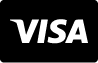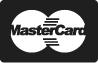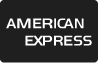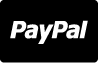Centrum Podpory

# Reklamní fotografie - Student doing math homework or mathematics test in school class. Writing mathematical equation and numbers for geometry assignment on paper with pen. Notes during lesson and teaching. Schoolwork.

Student doing math homework or mathematics test in school class. Writing mathematical equation and numbers for geometry assignment on paper with pen. Notes during lesson and teaching. Schoolwork.
ID obrázku : 117448286
Typ média :
Autorské právo :
7952 x 5304 px | 26.51 " x 17.68 " | 300dpi | JPG
Klíčová slova k fotografiím
Ukázat více
Show Less
Potřebujete poradit? Kontaktujte našeho zástupce na
1 866 655 3733